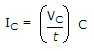The center frequency of a band-pass filter is always equal to the

The formulashows that for a given capacitor, if the voltage changes at a constant rate with respect to time, the current will

A digital-to-analog converter is an application of the

If the value of resistor Rf in an averaging amplifier circuit is equal to the value of one input resistor divided by the number of inputs, the output will be equal to

If the input to a comparator is a sine wave, the output is a

A basic series regulator has

A comparator is an example of a(n)

Initially, the closed-loop gain (Acl) of a Wien-bridge oscillator should be

In an averaging amplifier, the input resistances are

A zero-level detector is a